# 清泉知識課堂-汙水處理化學除磷知識

1、汙水中的磷負荷

2、化學除磷的基礎

FeCl3+K3PO4→FePO4↓+3KCl（式1）

3、化學除磷藥劑的類型

Al3++PO43-→AlPO4↓pH=6～7（式2）

Fe3++PO43-→FePO4↓pH=5～5.5（式3）

Al3++3OH-→Al(OH)3↓（式4）

Fe3++3OH-→Fe(OH)3（式5）

5Ca2++3PO43-+OH-→Ca5(PO4)3OH↓（式6）

Ca2++CO32-→CaCO3（式7）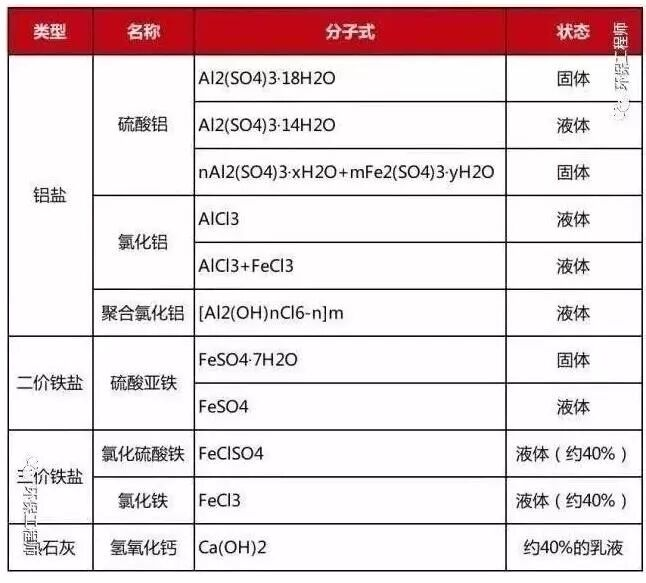4、化學沉析工藝

(1)前沉析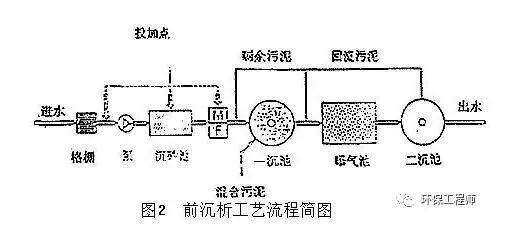(2)同步沉析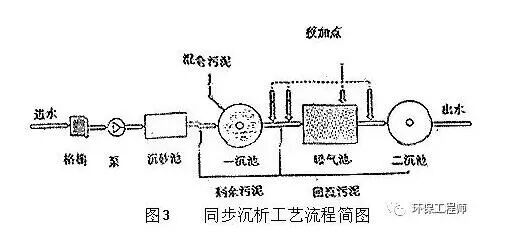(3)後沉析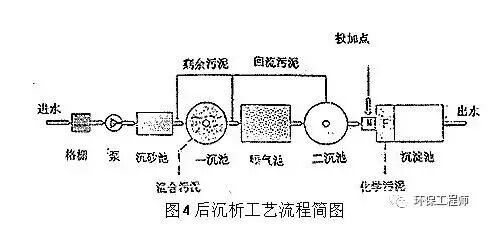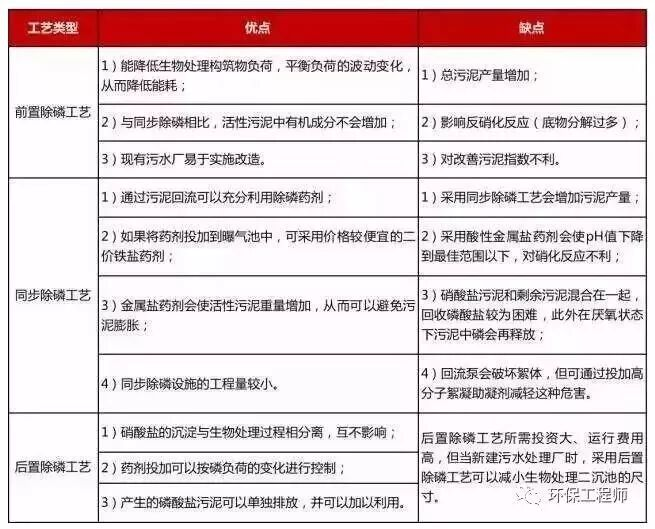5、化學沉析藥劑量的計算

β=(molFe,molAl)／molP（式8）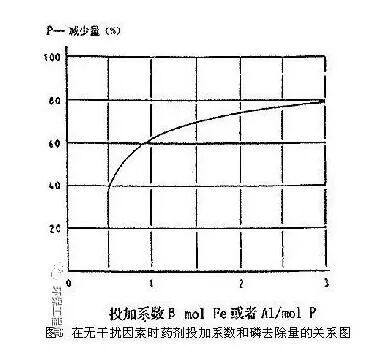1.5×（56／31）=2.7 kg Fe

1.5×（27／31）=1.3 kg Al

6、計算舉例

P負荷=

10000m3/d·(0.011-0.001)kg/m3=100kg/d

1.5×（27／31）×100=130kgAl/d

130×1000(g/d)/60(g/kg)=2167kg/dAlCl3

2167(kg/d)/1.3(kg/l)=16671/dAlCl3

1.5×5631×100=270kg Fe/d

270×1000(g/d)/180(g/kg)=1500kg/dFeSO4

180(g/kg)·0.4(kg/l)=72gFe/l FeSO4

1500·1000(g/d)/72(g/l)=20833l/dFeSO4

7、沉析對汙水處理的影響

(1)沉析對汙水處理廠出水金屬含量的影響

(2)沉析對出水中鹽含量的影響

130×3.94=513kgCl-/d

513kg/d×1000/10000m3/d=51.3mg/l

270×1.7=461.7kgSO24-/d

461.7kg/d×1000/10000m3/d=46.2mg/l

(3)沉析對堿度的影響

Me(H2O)63+→3H++Me(OH)3+3H2O（式9）

(4)沉析對剩餘汙泥產量的影響

(5)沉析對硝化反應的影響